Question

# We know that the magnitudes of the negative charge on the electron and the positive charge...

We know that the magnitudes of the negative charge on the electron and the positive charge on the proton are equal. Suppose, however, that these magnitudes differ from each other by 0.00060%. With what force would two copper coins, placed 0.76 m apart, repel each other? Assume that each coin contains 3.2 × 1022 copper atoms. (Hint: A neutral copper atom contains 29 protons and 29 electrons.)

q = difference of the charge between proton and electron = 0.000006 x 1.6 x 10-19 C

n = number of electrons or proton = 29

N = Number of atoms = 3.2 x 1022

Q = Total difference of charge = N n q = (3.2 x 1022) (29) (0.000006 x 1.6 x 10-19) = 0.89 C

r = distance between two coins = 0.76 m

Force between the coins is given as

F = k Q2/r2

F = (9 x 109) (0.89)2 /(0.76)2

F = 1.23 x 1010 N

#### Earn Coins

Coins can be redeemed for fabulous gifts.

Similar Homework Help Questions
• ### We know that the magnitudes of the negative charge on the electron and the positive charge...

We know that the magnitudes of the negative charge on the electron and the positive charge on the proton are equal. Suppose, however, that these magnitudes differ from each other by 0.00043%. With what force would two copper coins, placed 1.0 m apart, repel each other? Assume that each coin contains 4.3 × 1022 copper atoms. (Hint: A neutral copper atom contains 29 protons and 29 electrons.)

• ### We know that the negative charge on the electron and the positive charge on the proton...

We know that the negative charge on the electron and the positive charge on the proton are equal. Suppose, however, that these magnitudes differ from each other by 0.00012%. With what force would two copper pennies, placed 1.3 m apart, repel each other? Assume that each coin contains 3 ✕ 1022 copper atoms. (Hint: A neutral copper atom contains 29 protons and 29 electrons.) ________________N (From your answer, it should be obvious that the magnitude of the charge on a...

• ### Question 3-8 points To the best of our knowledge the magnitudes of the negative charge on...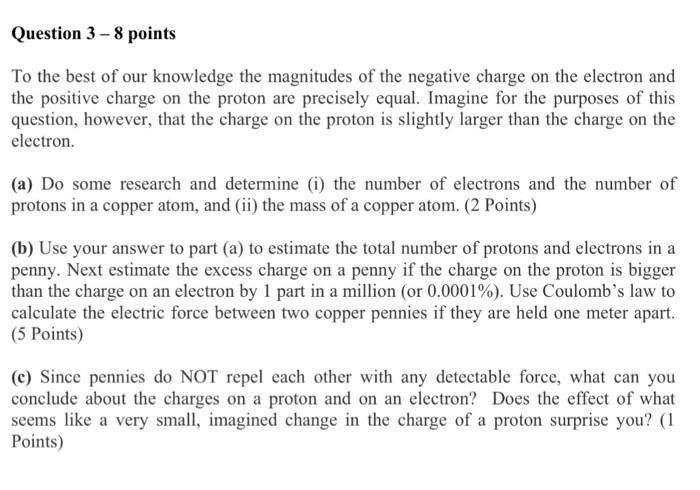Question 3-8 points To the best of our knowledge the magnitudes of the negative charge on the electron and the positive charge on the proton are precisely equal. Imagine for the purposes of this question, however, that the charge on the proton is slightly larger than the charge on the electron (a) Do some research and determine (i) the number of electrons and the number of protons in a copper atom, and (ii) the mass of a copper atom. (2...

• ### Suppose the magnitude of the proton charge differs from the magnitude of the electron charge by...

Suppose the magnitude of the proton charge differs from the magnitude of the electron charge by a mere 1 part in 10^9. A) What would be the force between two 1.1-mm-diameter copper spheres with 2.0 cm between their centers? Assume that each copper atom has an equal number of electrons and protons. Express your answer to two significant figures and include the appropriate units. B) Would this amount be detectable? C) What can you conclude from the fact that no...

• ### Question 1 What is the net electric charge (in units of e) of an atom of...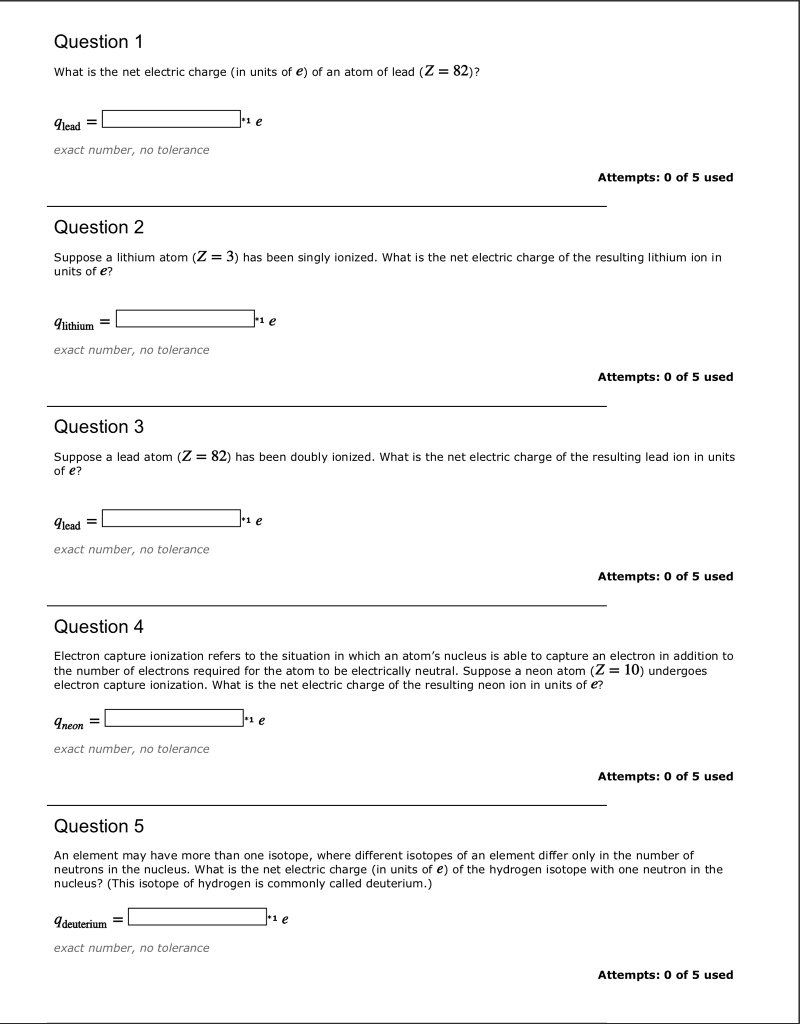Question 1 What is the net electric charge (in units of e) of an atom of lead (Z82)? qlead exact number, no tolerance Attempts: 0 of 5 used Question 2 Suppose a lithium atom (Z units of e? 3) has been singly ionized. What is the net electric charge of the resulting lithium ion in lithium exact number, no tolerance Attempts: 0 of 5 used Question 3 Suppose a lead atom (Z = 82) has been doubly ionized. What is...

• ### It is known that the magnitude of the charge on an electron and a proton are...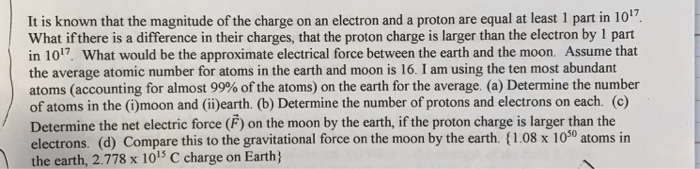It is known that the magnitude of the charge on an electron and a proton are equal at least 1 part in 1017 What if there is a difference in their charges, that the proton charge is larger than the electron by 1 part in 101. What would be the approximate electrical force between the earth and the moon. Assume that the average atomic number for atoms in the earth and moon is 16. I am using the ten most...

• ### A particular atom has 4 more protons than electrons. The atom has a net charge of...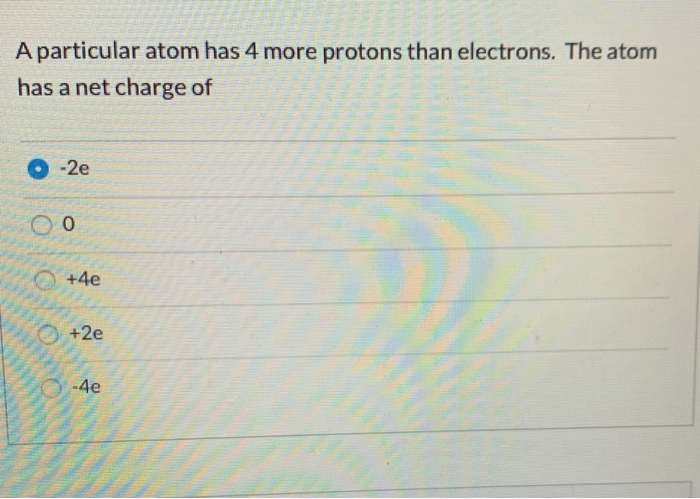A particular atom has 4 more protons than electrons. The atom has a net charge of O-2e oo +4e x2 Question 3 1 pts Which of the following is not a property of the electric force between charged objects? The direction of the force between charges is along the line from one charge to the other Charges of the same kind repel each other, and charges of different kinds attract All of the other choices are true properties of the...

• ### Atoms and Isotopes 1 Activity: Atoms and Isotopes Why? Atoms and isotopes are identified by the...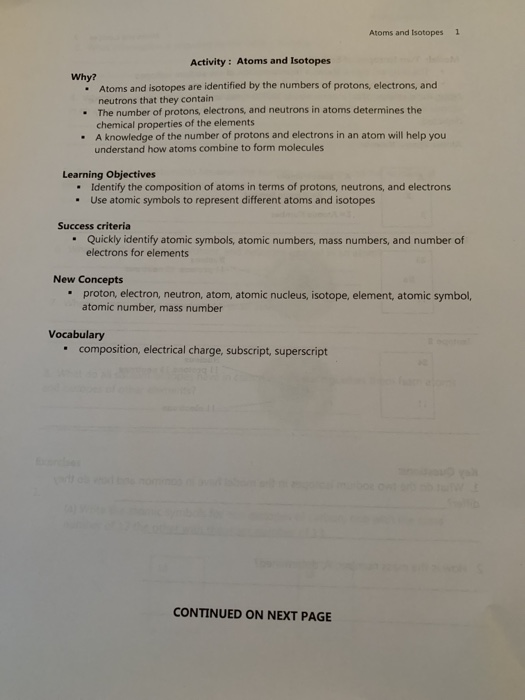Atoms and Isotopes 1 Activity: Atoms and Isotopes Why? Atoms and isotopes are identified by the numbers of protons, electrons, and neutrons that they contain The number of protons, electrons, and neutrons in atoms determines the chemical properties of the elements .A knowledge of the number of protons and electrons in an atom will help you understand how atoms combine to form molecules Identify the composition of atoms in terms of protons, neutrons, and electrons Use atomic symbols to represent...

• ### 1.-Discover the first sub - atomic particle ... the electron? 2.- The electrons move around the...

1.-Discover the first sub - atomic particle ... the electron? 2.- The electrons move around the nucleus , as do the planets around the sun 3.- If an electron absorbs energy can move to another orbit farther from the nucleus . 4.- Atoms are indivisible and differ in shape size , order and position 5.- Model based on the quantum numbers 6.- Explain the electrical nature of matter, why bodies attract or repel. 7.- Atom that is constituted by a...

• ### Effective Nuclear Charge and Periodic Trends Coulombs Law describes the interaction between two charges and varies...

Effective Nuclear Charge and Periodic Trends Coulombs Law describes the interaction between two charges and varies by the magnitude of these charges and inversely with the distance between them. ? ∝ ?1?2/? For atoms, we’ll label the charges as the nuclear charge and electron charge. ? ∝ ?????????/? As you go up in atomic number (Z), the number of protons in the nucleus increases, making the charge on the nucleus increase, so that in general. ???? = ? ∙ (+1)...